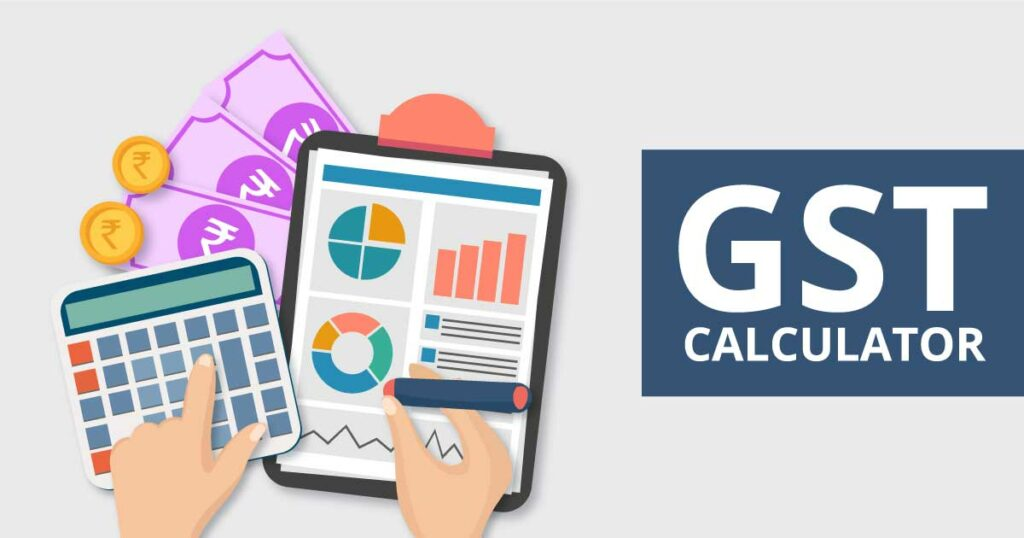# GST Made Easy: Simplifying Tax Calculations with the Formula for Businesses

In India, Goods and Services Tax (GST) is a critical aspect of taxation and a valuable tool for promoting the ease of doing business. Accurate GST calculations are an important part of managing tax liabilities for businesses, and failure to comply can lead to serious financial and legal implications.

The GST calculation formula is the basic tool for businesses to calculate their GST liabilities correctly. In this article, we will simplify tax calculations with the formula for businesses and highlight the benefits of using GST interest calculators to streamline the tax calculation process.

## Understanding the GST Calculation Formula

The GST calculation formula is relatively straightforward and comprises two elements: the taxable value and the GST rate. The formula for the calculation of GST is:
GST Amount = (Taxable Value * GST Rate) / 100

Where:

– Taxable value is the amount that is liable to GST
– GST rate is the tax rate applicable to the transaction

To illustrate the calculation process, let us consider the following scenario:

Scenario: A business owner sells goods worth INR 10,000, and the GST rate is 5%. How much GST does the business owner need to charge?

GST Amount = (10,000 * 5)/100 = INR 500

Therefore, the business owner needs to charge a GST of INR 500.

## Benefits of Simplifying Tax Calculations with GST Calculation Formula

Simplifying tax calculations with the GST calculation formula has many benefits for businesses, including:

1. Accurate calculations: The GST calculation formula is simple and easy to use, enabling businesses to calculate their tax liabilities accurately and avoid any potential penalties.

2. Time-saving: The GST calculation formula is quick to use, saving businesses time and allowing them to focus on other essential activities.

3. Cost-effective: The GST calculation formula does not require businesses to pay for expensive software or outside assistance, reducing operational costs and maximizing profits.

4. Improved Compliance: Accurately calculating GST liabilities and making prompt payments enhance business compliance, enabling companies to avoid any legal or financial implications.

### Using GST Interest Calculators to Streamline Tax Calculations

In addition to using the GST calculation formula, businesses can also benefit from using GST interest calculator. GST interest calculators aid business owners in determining the accurate interest payable on delayed tax payments. These online calculators are designed to save businesses time and money by automating the process of computing GST interest amounts.

To use the GST interest calculator, businesses only need to input the principal amount and the number of days the payment has been delayed. These online calculators use the GST interest formula to compute the interest for businesses accurately. The GST interest formula is:

Interest = (principal amount x rate of interest x time period) / 365

Where:

– Principal amount is the outstanding amount of tax
– The rate of interest is 18% per annum
– The time period is calculated on a daily basis

Using GST interest calculators can help businesses calculate the correct GST liabilities and avoid any costly errors.

## Conclusion

GST is an essential part of business taxation in India. Effectively managing GST obligations is crucial to the financial success of a business. The GST calculation formula is a useful tool for calculating business tax obligations accurately and efficiently. Utilizing GST interest calculators can streamline the tax calculation process and reduce the risk of human error.

Using these calculators and simplifying tax calculations with the GST calculation formula can help businesses save time, reduce costs, and improve compliance. Taxation experts in India recommend leveraging the latest technology and online solutions to stay compliant with GST laws and regulations. By doing so, businesses can minimize tax liabilities, reduce penalties, and optimize profits, causing them to stay ahead in the competitive market.